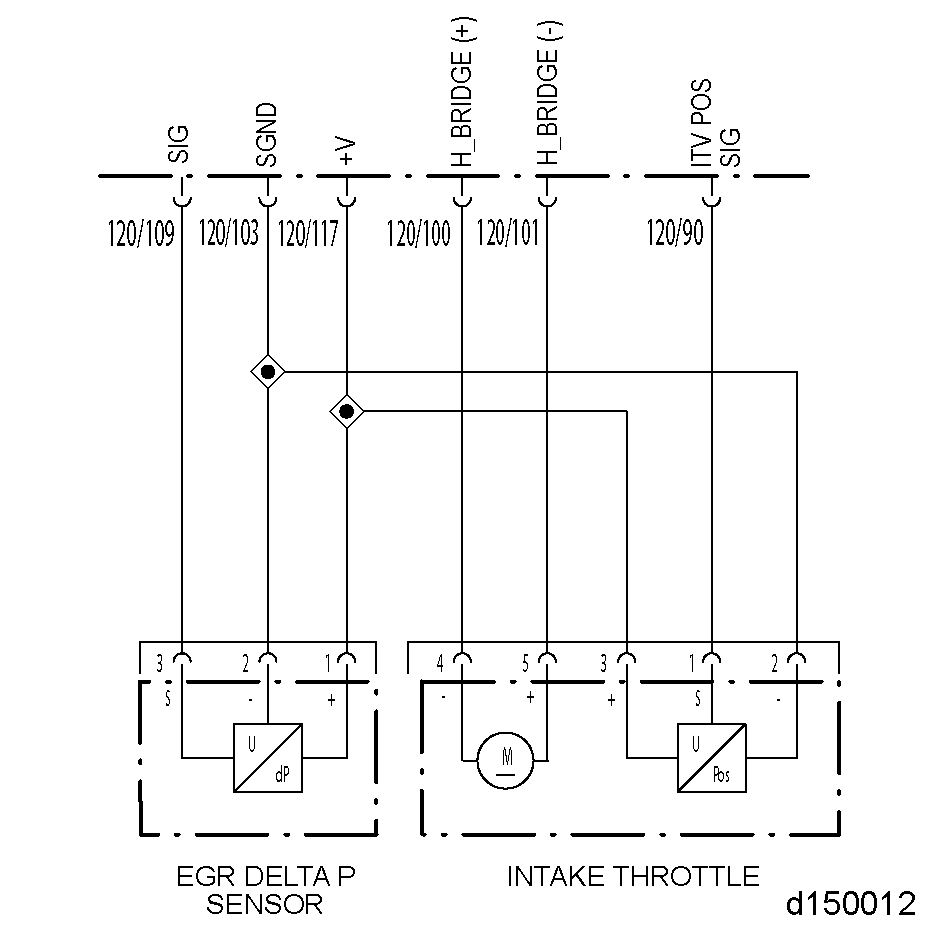# Section 39.3 SPN 411/FMI 3

### Section 39.3 SPN 411/FMI 3

This diagnosis is typically EGR Delta P Sensor circuit failed high.‪Check as follows:‪

1. Disconnect the EGR Delta P Sensor.
2. Measure the resistance across pins 1 and 3 of the EGR Delta P Sensor.
1. If the resistance is greater than 130 kΩ, replace the EGR Delta P Sensor. Refer to "39.3.1 Verify Repairs" .
2. If the resistance is less than 130 kΩ, go to the next step.
3. Measure the resistance between pins 1 and 2 of the EGR Delta P Sensor.
1. If the resistance is greater than 130 kΩ, replace the EGR Delta P Sensor. Refer to "39.3.1 Verify Repairs" .
2. If the resistance is less than 130 kΩ,, go to the next step.
4. Turn the ignition ON (key ON, engine OFF).
5. Measure the voltage between pins 1 and 2 of the EGR Delta P Sensor harness connector.
1. If the voltage is between 4.5 and 5.5 volts, go to step 7 .
2. If the voltage is less than 4.5 volts, go to the next step.
6. Measure the voltage between pin 1 of the EGR Delta P Sensor harness connector and ground.
1. If the voltage is between 4.5 and 5.5 volts, repair the open circuit between pin 2 of the EGR Delta P Sensor harness connector and pin 103 of the 120–pin MCM connector. Refer to "39.3.1 Verify Repairs" .
2. If the voltage is less than 4.5 volts, repair the open circuit between pin 1 of the EGR Delta P Sensor harness connector and pin 117 of the 120–pin MCM connector. Refer to "39.3.1 Verify Repairs" .
7. Turn the ignition OFF.
8. Disconnect the 120–pin MCM connector.
9. Measure the resistance between pins 1 and 3 of the EGR Delta P Sensor harness connector.
1. If the resistance is less than 5 Ω, repair the short in wires between pins 1 and 3 of the EGR Delta P Sensor harness connector and pins 117 and 109 of the 120–pin MCM connector. Refer to "39.3.1 Verify Repairs" .
2. If the resistance is greater than 5 Ω, go to the next step.
10. Measure the resistance across pins 2 and 3 of the EGR Delta P Sensor harness connector.
1. If the resistance is less than 5 Ω, repair the short in wires between pins 109 and 103 of the 120–pin MCM connector. Refer to "39.3.1 Verify Repairs" .
2. If the resistance is greater than 5 Ω, go to the next step.
11. Measure the resistance between pin 1of the EGR Delta P Sensor harness connector and ground.
1. If the resistance is less than 5 Ω, repair the short circuit between pin 117 of the 120–pin MCM connector and ground. Refer to "39.3.1 Verify Repairs" .
2. If the resistance is greater than 5 Ω, go to the next step.
12. Measure the resistance between pin 3 of the EGR Delta P Sensor harness connector and ground..
1. If the resistance is less than 5 Ω, repair the short between pin 109 of the 120–pin MCM connector and ground. Refer to "39.3.1 Verify Repairs" .
2. If the resistance is greater than 5 Ω, repair the open circuit between pin 3 of the EGR Delta P Sensor harness connector and pin 109 of the 120–pin MCM connector. Refer to "39.3.1 Verify Repairs" .

#### Section 39.3.1 Verify Repairs

Verify repairs as follows:‪

1. Turn ignition OFF.
2. Reconnect any electrical connections that were disconnected to perform the diagnosis.ENGINE EXHAUST ‪ To avoid injury from inhaling engine exhaust, always operate the engine in a well-ventilated area. Engine exhaust is toxic.‪